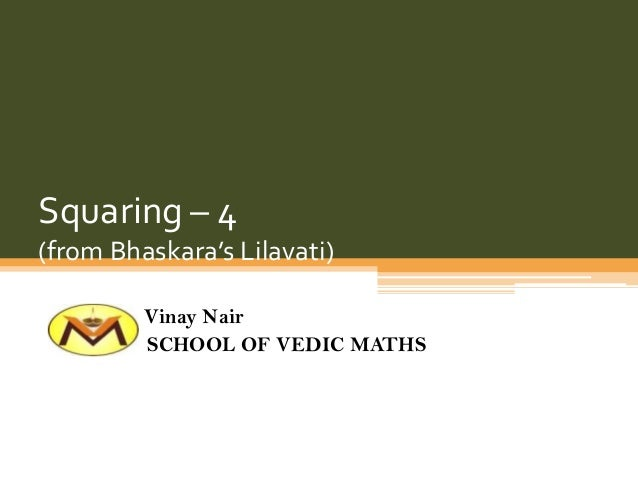Successfully reported this slideshow.
We use your LinkedIn profile and activity data to personalize ads and to show you more relevant ads. You can change your ad preferences anytime.Upcoming SlideShare
×

# Squaring 4

506 views

Published on

An intelligent way of squaring numbers using an algebraic identity given by ancient Indian mathematician Bhaskaracharya-II (1114-1193 CE). Learn this technique to do squaring mentally.
Teachers can use this technique to teach mental squaring.

Published in: Spiritual, Education
• Full Name
Comment goes here.

Are you sure you want to Yes No
Your message goes here• Be the first to comment

• Be the first to like this

### Squaring 4

1. 1. Squaring – 4 (from Bhaskara’s Lilavati) Vinay Nair SCHOOL OF VEDIC MATHS
2. 2. • a2 – b2 = (a + b)(a – b) • a2 = (a + b)(a – b) + b2
3. 3. a2 = (a + b)(a – b) + b2 (i) 462 = (46 + 4)(46 – 4) + 42 = 50 x 42 + 16 = 2100 + 16 = 2116 (ii) 582 = (58 + 8)(58 – 8) + 82 = 66 x 50 + 64 = 3300 + 64 = 3364
4. 4. a2 = (a + b)(a – b) + b2 (iii) 382 = (38 + 2)(38 – 2) + 22 = 40 x 36 +4 = 1440 +4 = 1444 (iv) 272 = (27 + 3)(27 – 3) + 32 = 30 x 24 + 9 = 720 +9 = 729
5. 5. a2 = (a + b)(a – b) + b2 (v) 932 = (93 + 7)(93 – 7) + 72 = 100 x 86 + 49 = 8600 + 49 = 8649 (vi) 892 = (89 + 11)(89 – 11) + 112 = 100 x 78 + 121 = 7800 + 121 = 7921
6. 6. Thank you!